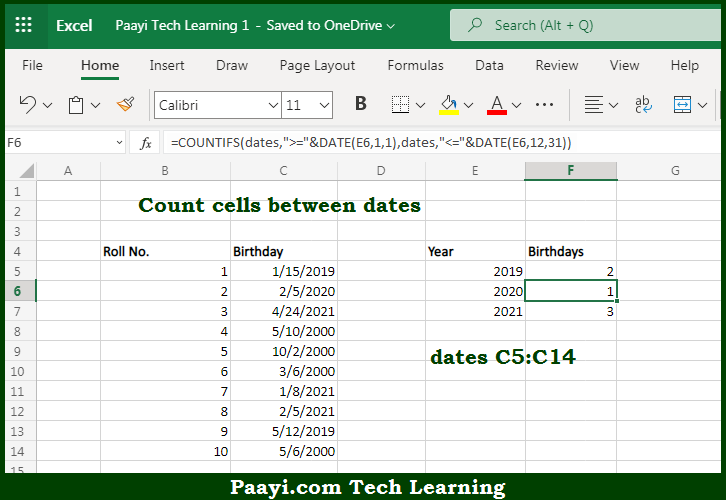# Learn How to Count Cells Between Dates in Microsoft Excel

Written by | 0 Comments | 543 Views

In this article, you will learn how to use the Microsoft Excel COUNT formula and its purpose in Microsoft Excel. You will also get to know how to Count Cells Between Dates and see the generic formula.

Count Cells Between Dates Using Microsoft Excel Count Formula

The main purpose of the Microsoft Excel COUNT formula is to count the number of cells that contain dates between two dates. Here we will learn how to count cells between two dates.  That implies, with the help of the COUNT formula you can able to count the number of cells that contain dates between two dates, It should be noted that you can use the COUNTIFS function. So, with the help of the COUNT formula, you can able to count the number of cells that contain dates between two dates.

General Syntax of the COUNT Formula To Count Cells Between Dates

=COUNTIFS(range,">="&date1,range,"<="&date2)

### Explanation for the Count Cells Between DatesSo we know that Microsoft Excel COUNT formula you can able to count the number of cells that contain dates between two dates. Here we will learn how to count cells between two dates. The COUNTIFS function is used to count cells that meet multiple criteria. In the example given above, we have provided two criteria, one criterion for the earlier date and one for the later date. So, with the help of the COUNT formula, you can able to count the number of cells that contain dates between two dates.